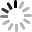2．一堆煤重600t，第一次运走总数的$\frac{1}{3}$，第二次比第一次多运走20%，这堆煤还剩多少吨？

=600×[$\frac{2}{3}-\frac{1}{3}×\frac{6}{5}$]
=600×[$\frac{2}{3}-\frac{2}{5}$]
=600×$\frac{4}{15}$
=160（吨），